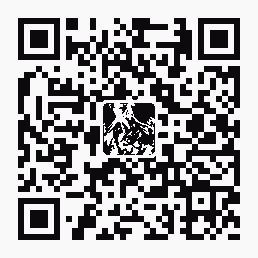| 導購 |订阅 | 在线投稿

# 透徹掌握ASP分頁技術

2008-09-18 07:06:50  編輯來源:互聯網  简体版  手機版  移動版  評論  字體: ||

<%

Set conn=Server.CreateObject("Adodb.Connection")

connstr="provider=Microsoft.JET.OLEDB.4.0;Data Source="&Server.MapPath("data.mdb")

conn.open connstr

Set rs=Server.CreateObject("Adodb.Recordset")

sql="Select * from info"

rs.open sql,conn,1,1

%>

<%

rs.pagesize=5

curpage=Request.QueryString("curpage")

if curpage="" then curpage=1

rs.absolutepage=curpage

%>

<%

for i= 1 to rs.pagesize

if rs.eof then

exit for

end if

%>

<%=rs("record_info")%><br>

<%

rs.movenext

next

%>

for循環語句。

<%if curpage=1 then%>

<%else%>

<a href="?curpage=1">首頁</a>

<%end if%>

<%if curpage=1 then%>

<%else%>

<a href="?curpage=<%=curpage-1%>">上一頁</a>

<%end if%>

<%if rs.pagecount<curpage+1 then%>

<%else%>

<a href="?curpage=<%=curpage+1%>">下一頁</a>

<%end if%>

<%if rs.pagecount<curpage+1 then%>

<%else%>

<a href="?curpage=<%=rs.pagecount%>">尾頁</a>

<%end if%>

󰈣󰈤

>>返回首頁<<

熱帖排行© 2005- 王朝網路 版權所有